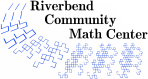# Candy Sharing Game

### Description

Students learn a candy sharing game with a simple rule for passing candy around the circle. Students will experiment to figure out how to make the game stop, end in a fixed point, or end in a cycle depending on the amount of starting candy, the number of people playing, and the initial distribution of candy.

### Levels

• This activity can be extended to an undergraduate research project.

5 minutes

### Activity Time

25 to 60 minutes for the introductory activity. It is possible to extend this into a sequence of classes relating to matrices or dynamical systems.

### Topics

• Dynamical Systems
• Number Patterns

### Goals

• Students will learn what a dynamical system is.
• Students will learn the terms fixed point and cycle.
• Students will find an initial distribution of candy that causes the game to stop.
• Students will find an initial distribution of candy that leads to a fixed point.
• Students will find an initial distribution of candy that leads to a cycle.
• Students will consider the amount of candy they need to continue their game forever.
• Students will consider the largest amount of candy they can have in a game that leads to a cycle instead of a fixed point.

### Materials

• Paper for making notes.
• Pencils.
• Wrapped candies (4 to 10 for every student).
• Challenges Handouts for each group of 3 to 6 students.
• An easel, blackboard, or whiteboard with markers or chalk.

### Prerequisites

• Counting

• Matrix operations

### Related Concepts

• Markov chains
• Dynamical systems

### Credits

Boston Math Circle research page
http://www.themathcircle.org/researchproblems.htm
Pass the Candy -- An Introduction to Recursive Equations. Maria Hernandez, NCSSM
courses.ncssm.edu/math/TCMConf/TCM2004/TCMTalks/CandyLong.pdf

### National Common Core Standards

 HS.Modeling Modeling is the process of choosing and using appropriate mathematics and statistics to analyze empirical situations, to understand them better, and to improve decisions. Quantities and their relationships in physical, economic, public policy, social, and everyday situations can be modeled using mathematical and statistical methods. When making mathematical models, technology is valuable for varying assumptions, exploring consequences, and comparing predictions with data. HS.N-VM.6 Use matrices to represent and manipulate data, e.g. to represent payoffs or incidence relationships in a network. HS.N-VM.11 Multiply a vector (regarded as a column matrix) by a matrix of suitable dimensions to produce another vector. Work with matrices as transformations of vectors.
Riverbend Community Math Center
hello@riverbendmath.org
http://riverbendmath.org
(574) 339-9111This work placed into the public domain by the Riverbend Community Math Center.